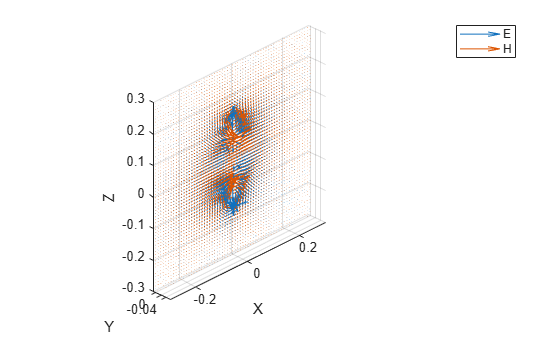# Custom Radiation Pattern and Fields

This example shows how to visualize a radiation pattern and vector fields from the user imported pattern data. Use the `patternCustom` function to plot the field data in 3-D. This function also allows you to view the sliced data. Alternatively, use the `polarpattern` object to visualize the field data in 2-D polar format. The `polarpattern` function allows you to interact with the data and perform antenna specific measurements. You can also plot the vector fields at a point in space using the `fieldsCustom` function.

### Import 3D Pattern Data

Use the `readmatrix` function to read the 3D Radiation pattern data stored in a `.csv` file. In the first part of this example, you use the `patternCustom` function to visualize the 3D data. The function can also be used to visualize 2D slices of the 3D data.

`M = readmatrix("CustomPattern_testfile.csv");`

### Plot 3D Radiation Pattern on Polar Coordinate System

Specify the electric field magnitude (MagE) vector/matrix along with the theta and phi vectors to plot the 3D radiation pattern on a polar coordinate system. If MagE is a matrix, it must be of size phi-by-theta. If MagE is a vector, then all the 3 arguments MagE, phi and theta must be of the same size.

`patternCustom(M(:,3),M(:,2),M(:,1));`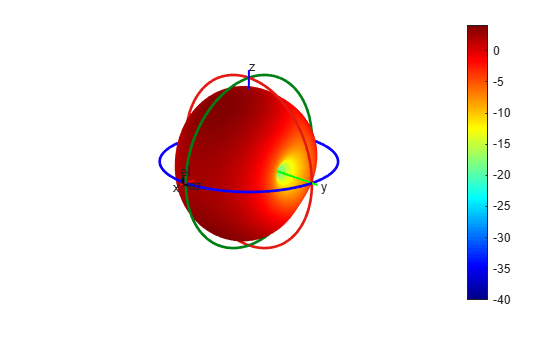### Plot 3D Radiation Pattern on Rectangular Coordinate System

Specify the `CoordinateSystem` property as "rectangular" to plot the 3-D radiation pattern on a rectangular coordinate system. The default coordinate system is polar.

`patternCustom(M(:,3),M(:,2),M(:,1),CoordinateSystem="rectangular");`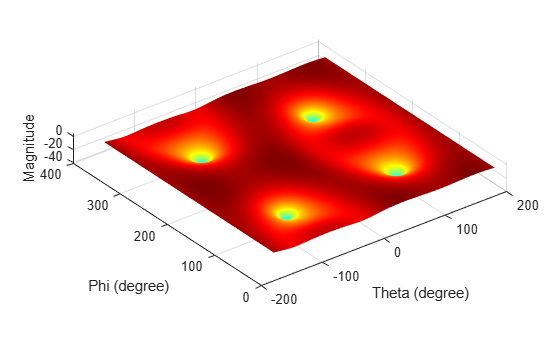### Visualize 2D Slices from the 3D Data

Specify the `CoordinateSystem` property as "polar" to plot a 2-D slice on a polar coordinate system plot. Specify the `Slice` property to either "phi" or "theta", depending on the plane you want to view the data in. Additionally, specify the `SliceValue` property with a vector of phi or theta angles for the slices as input values.

```patternCustom(M(:,3),M(:,2),M(:,1),CoordinateSystem="polar",... Slice="phi",SliceValue=[45 90 180 360]);```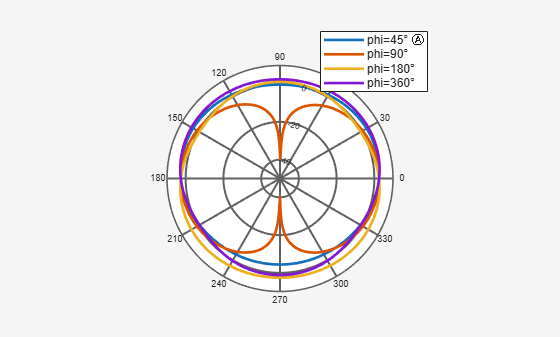Set the `CoordinateSystem` property to "rectangular" to plot the same data in a rectangular coordinate system plot.

```patternCustom(M(:,3),M(:,2),M(:,1),CoordinateSystem="rectangular",... Slice="phi",SliceValue=[45 90 180 360]);```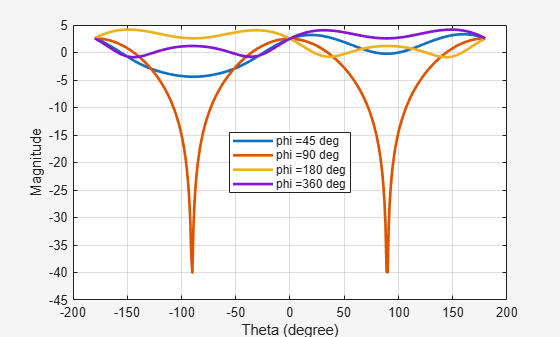### Plot 2D Polar Data

Use the `polarpattern` function to plot pattern data in polar format as shown below. The generated plot is interactive and allows you to perform antenna specific measurements. The data for this illustration is stored in a MAT file which stores directivity values calculated over 360 degrees with one degree separation.

```load polardata p = polarpattern(ang, D);```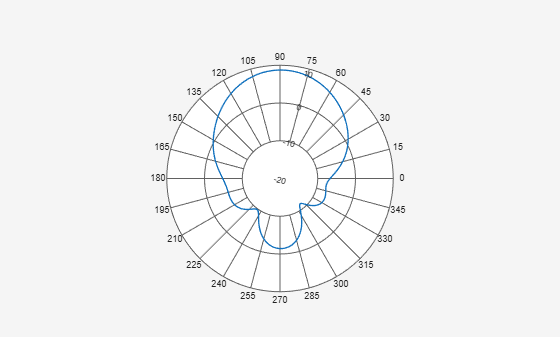Right-click inside the figure window to interact with the plot. The figure below shows a screen shot of the context menu. Context menus can be used to do measurements such as peak detection, beamwidth calculation etc. You can also add a cursor by right clicking inside the polar circle.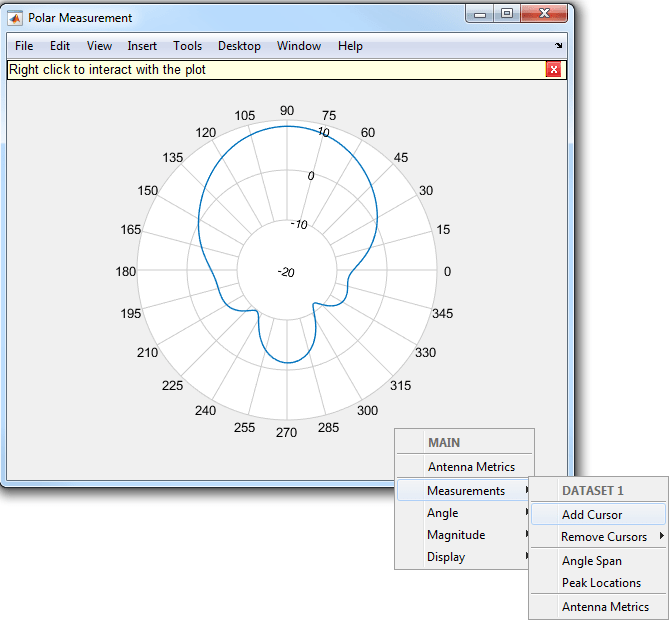Select the Antenna Metrics option in the context menu shown above, to visualize the antenna specific measurements as shown below.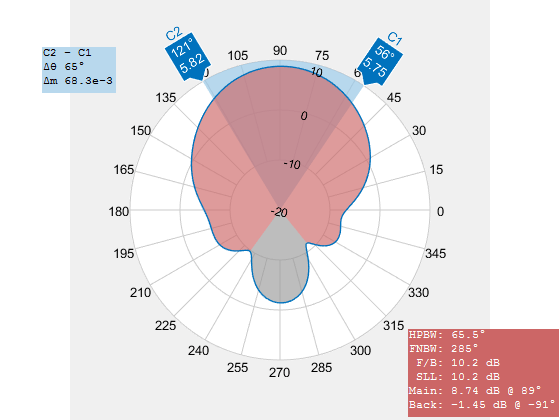### Plot Vector Field Data at a Point in Space

Use the `fieldsCustom` function to plot vector electric and/or magnetic fields at any point in space as shown below. The MAT file `EHfielddata` contains the E and H field data at the points in space specified by the x, y and z coordinates. The electric and magnetic fields are complex quantities and have x, y and z components at every point in space. The fields can be artificially scaled for better visualization.

```load EHfielddata; figure fieldsCustom(H, points, 5);```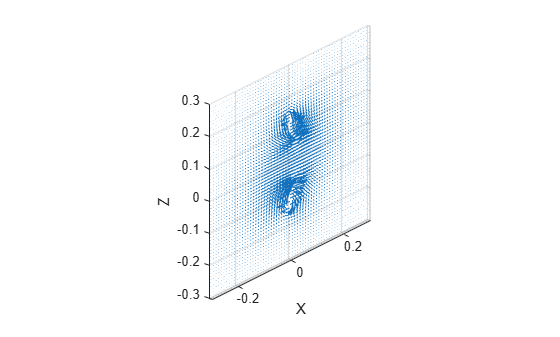The function is used to plot one field quantity at a time. To plot both E and H fields on the same plot, use the hold on command.

```figure fieldsCustom(gca, E, points, 5); hold on; fieldsCustom(gca, H, points, 5); hold off; legend("E","H");```